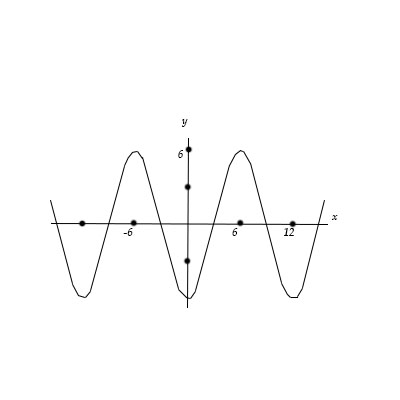# The graph of a sine function with a positive coefficient is shown in the figure below. a. Find...

## Question:

The graph of a sine function with a positive coefficient is shown in the figure below.

a. Find the amplitude, period and phase shift.

b. Write the equation in the form {eq}y=a \sin (bx-c) {/eq}for {eq}a>0,b>0 {/eq}, and the least positive real number {eq}c {/eq}.## Sinusoidal Function:

A sinusoidal function is a function defined by the following relation

{eq}y=a \sin (bx-c) {/eq}

where a is the amplitude, {eq}2\pi/b {/eq} is the period and c is the phase shift.

We are given the graph of a sine function

{eq}y=a \sin(bx-c) {/eq}

where a is the amplitude, {eq}\frac{2\pi}{b} {/eq} is the period and {eq}c {/eq} is the phase shift.

From the graph we see that the amplitude is

{eq}a=6 {/eq}

the period is

{eq}\displaystyle \frac{2\pi}{b} = 12 \Rightarrow b = \frac{\pi}{6} {/eq}

and the phase shift is

{eq}\displaystyle c=3. {/eq}

Therefore, the function is written as

{eq}y=6 \sin(\frac{\pi}{6}x-3) {/eq}